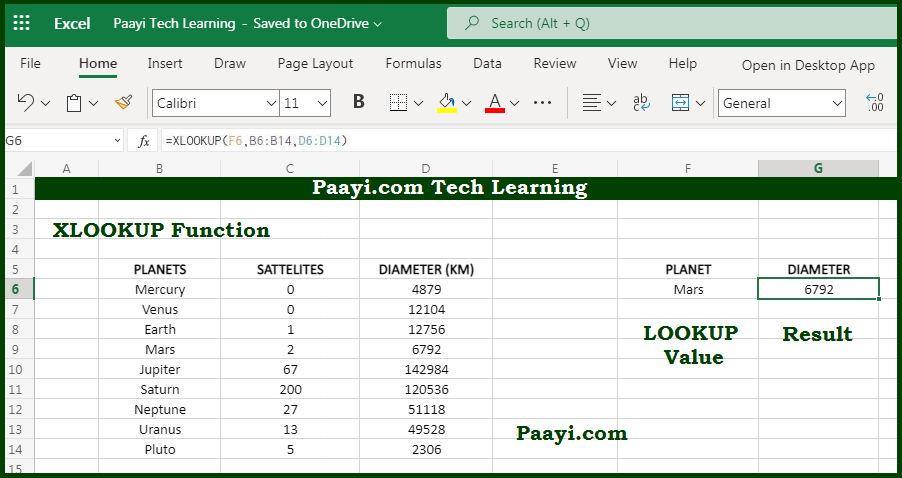# Learn How to Use Microsoft Excel XLOOKUP Function

Written by | 0 Comments | 636 Views

In this article, you will learn how to use the Microsoft Excel XLOOKUP function and its prime function in Microsoft Excel. You will also get to know the Microsoft Excel XLOOKUP function return value and syntax with the help of some examples.

Microsoft Excel XLOOKUP Function

The primary function of the Microsoft Excel XLOOKUP function is lookup values in a range or array. The XLOOKUP function is modern and flexible than its other counterparts like - VLOOKUP, HLOOKUP, and LOOKUP. The XLOOKUP function supports approximate and exact matching, wildcards for partial matching, and lookups in horizontal and vertical ranges.

Return Value of XLOOKUP Function

The return value will be the matching values from the return array.

Syntax of XLOOKUP Function

=XLOOKUP(lookup, lookup-array, return-array, [not-found], match-mode], [search-mode])

Where the arguments:

lookup: This is the lookup values.

lookup-array: This is the array or range to search.

return-array: This is the array or range to return.

not-found: This is the value to return if no match is found (optional).

match-mode: 0 = exact match (default), -1 = exact match or next smallest, 1 = exact match or next larger, 2 = wildcard match (optional).

search-mode: 1 = search from first (default), -1 = search from last, 2 = binary search ascending, -2 = binary search descending (optional).

## How to Use Microsoft Excel XLOOKUP Function?The XLOOKUP function is the modern replacement of the VLOOKUP function; it is more flexible and versatile and used in various situations. The XLOOKUP function can find the values in horizontal and vertical ranges, perform approximate and exact matches, and support wildcards for partial matches.

Match Type                           Behavior

0 (default)                                 Exact match, and return #N/A if no match

-1                                             Exact match or next smaller item

1                                              Exact match or next larger item

2                                              Wildcard match

Search Mode                          Behavior

1 (default)                              Search from the first value

-1                                             Search from last value

2                                              Binary search values sorted in an ascending order

-2                                             Binary search values sorted in the descending order